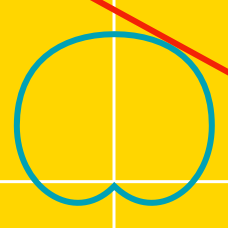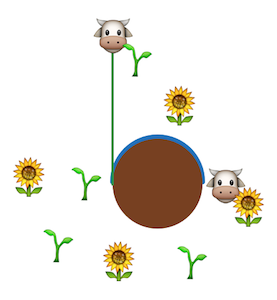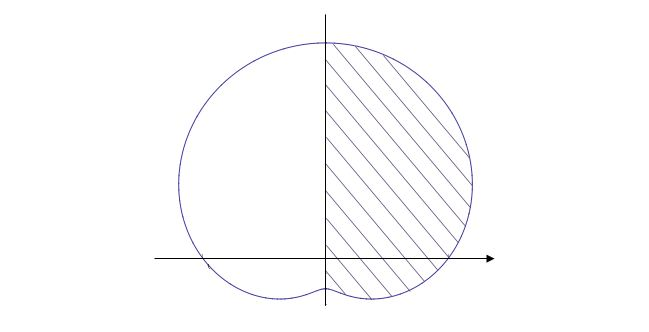Calculus

# Polar Equations - AreaA cow is tied to one point of a silo of radius 30, with a rope of length $30 \pi$ which would allow it to reach the opposite end of the silo.

What is the total area of the grass that the cow can graze on?Given the graph above of the polar equation $r=4+3\sin \theta,$ what is the area of the shaded region?

Find the area enclosed by one loop of the $4$-petal flower defined by the parametric equation $r=5\cos 2\theta.$

Find the area of the region that lies inside the circle $r=27\sin \theta$ and outside the cardioid $r=9+9\sin \theta.$

Find the area of the region bounded by the curve defined by $r^2=5\sin 2\theta, r \geq 0, 0 \leq \theta \leq \frac{\pi}{2}.$

×